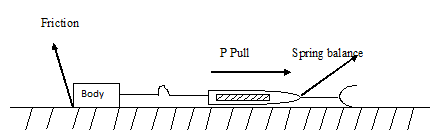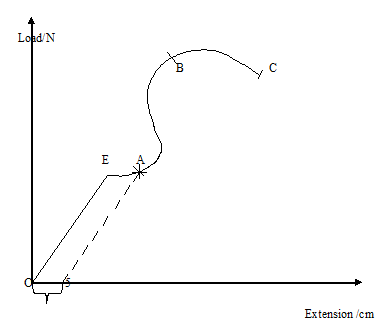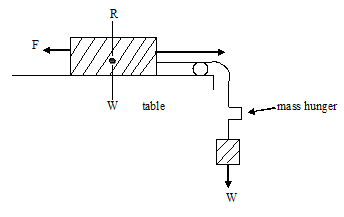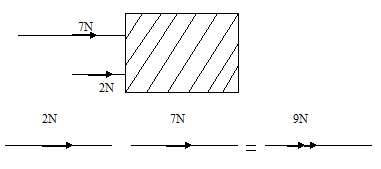# PHY1: FRICTION

##### This unit is about friction and the various calculations involved in it.

FRICTION

What is the frictional force?

Frictional force is the force which opposes the relative sliding motion of two surfaces in contact with one another.

What causes friction?

When two or more moving substances get into contact.

List the effects of friction

– Friction can lead to unnecessary fires

– Frictions wear out tyres of vehicles

– It wears out machines

– It enables us to write on paper

How can friction be reduced?

– Oiling/ greasing rough and objects

– Using roller or ball bearings

– By smothering

– Using rough stones e.g on roads

– It helps in writing using a paper and a pen

– It helps in movement

– It helps in lighting using match stick

– It prevents cars or people from falling

– It produces unnecessary noise

– It causes unnecessary heart which may cause fires

– It wears out objects e.g. soles of shoes, tyres e.t.c

TYPES OF FRICTION

Static:

This is friction experienced between two surfaces both stationary but one just at the point of slipping.

Dynamic

This is the type of friction experienced between two moving surfaces with a uniform relative velocity.

FACTORS THAT AFFECTS FRICTION

Nature of the surface

– attraction between molecules

– The weight of the body i.e. the behavior the object the more friction

FACTORS THAT DO NOT AFFECT FRICTION

– The speed of object

– The surface area with the area of contact

LAWS OF FRICTIONS

1. Frictional force between two surfaces opposes their relative motion.
2. The friction force is independent of the area of contact with the given surface when the normal reaction is constant.NOTE: Action and reaction forces are equal i.e. R = Mg

1. Frictional force is proportional to the normal reaction [R] i.e.

F µ R

F= Ω R

MEASUREMENTS OF FRICTIONWhen a gradually increasing force P is applied and a body is attached to the spring it does not move when it has just been fixed.

It is because an equally opposing force is acting where the body and Table are attached.

When P is moved further the body moves, at this point the friction is at its maximum value called static friction.

ExperimentDYNAMIC FRICTION

The difference is the weights [masses] are added to the mass hunger and a small push given until the block moves at a uniform velocity

LIMITING FRICTIONAL FORCE

This is the force between two different surfaces just before they slip from each other

THE FORCE OF ELASTICITY

This is the force that enables a material to stretch and return to its original size when the force is removed.

HOOKER’S LAW OF ELASTICITY

It states that the extension of an elastic material is directly proportional to the force causing it provided the elastic limit is not exceeded ie;-

F e

F = k e

Where k is the constant for proportionality

EXAMPLES

Given that when a force of 5N is human on a string it extends by 10mm.

Find the constant of proportionality of the spring

F = 5N, e = 10mm

Since F = k e

5/10    = k x 10/10

K= 0.5n/mm

For every 0.5n used, it is extended by 1mm

What force will extend the spring by 6mm?

F= k e

=0.5 x 6

= 3N

What mass will be needed to extend the spring by 12mm?

F = k e

= 0.5 x 12

= 6N

F= mg

6N = m x 10

6n/10  = 10m/10

M =6/10

M =3/5

M = 0.6kg

1. A spring 4cm long stretches to 7cm when a force of 15N is hang on it.

Find;-

1. The proportionality constant

F = 15N,   e=7cm-4cn=3cm.

Since f = k e

15n/3 = k x 3/3

= 5N/cm

What force will extend the spring by 6cm

F= ke

= 5 x 6

= 30N

What mass will be needed to extend the spring to 12cm

F =,    e = 12-4

8cm

F= k e

= 5 x 8

= 40N

F = mg

40/10  = m x 10/10

M= 4kg

What length will the spring be if a mass of 2.5 kg is hang on it.

F= mg

= 2.5 x 10

= 25N

THE GRAPH OF FORCE AGAINST EXTENSIONOE is a straight line passing through the origin. In this region, the spring is elastic it stretches when force is extended on it and returns to its original length when the force is removed. Hooker’s law is obeyed here.

Point A is called the yield point and as is the permanent extension. It is obtained when the spring does not return to its original length. It will have acquired a permanent extension.

Point E is the elastic limit

A is the yield point

Between A and B we have a region of plastic deformation. The spring behaves like a plastic.

Beyond A and B the extension increases rapidly when a force is added to it before eventually bears at C i.e. point C is the breaking point.

Measurement of frictionIn the experiment, known mass are added to the mass hanger in bite with the wooden block moves off with uniform velocity. When this happens, the weight on the mass hanger and mass hunger is equal to the maximum friction force [F] also called the limiting friction.

DYNAMIC/SLIDING FRICTION

The same arrangement as above is set up. The difference is that when masses are added to the mass hanger, small push is given to the wooden block. This is done until he black moves with a uniform velocity.

MEASUREMENT OF FRICTION

A gradually increasing force P is applied on the body connected to the spring balance.When a gradually increasing force P is applied and a body is attached to the spring it does not move when it has just been fixed.

At first it does not move. This is because equally increasing but apposing frictional force [F] acts where the body and table touch at an instant. P and f are equal but opposite

If P is increased further, the body moves. At this point, F has its maximum value called the starting /static friction.

When the body is moving at a steady speed [uniform velocity] the balance reading is slightly less than that recording for the starting friction. Therefore kinetic friction is less than static friction.

When carrying out experiments investigation the extension and force that causes it. [hookes low]

Values of mass in grams and the corresponding lengths of the strings are normally recorded in a table

Because we are interested in the force load and the corresponding extension, this table of results is normally modified to give what is required.

EXAMPLES

1. If in an experiment the following values of mass and the corresponding values of the length of the spring are shown in the table below. Find values of force and the corresponding extensions.
 Mass [g] 0 10 20 30 40 50 60 Length [cm] 4 4.2 4.4 4.5 4.8 5.1 5.2

Solution

 MASS LENGTH [CM] FORCE [N] EXTENSION [CM] 0102030405060 4.24.44.54.85.15.2 0.10.20.30.40.50.6 0.00.20.40.50.81.11.2

VECTORS AND SCALARS

Vectors

Quantities fully defined by magnitude and direction e.g;-

– Acceleration

– Velocity

– Weight

– Displacement

– Work

Scalars

Quantities fully defined by magnitude e.g.-

– Mass

– Temperature

– Distance

– Time

– Volume

– Speed

– Area

– Density

We normally use straight lines and arrows to represent vectors. The length of the line gives the magnitude where the arrows give the direction of the vector e.g.

1. 2RM
2. 2M
3. 2M
4. 3M

Vectors A and C are equal because are to the same direction.

We use ordinary arithmetic to add or subtract scalar quantities e.g. if a mass of two kilograms [2kg] is added to a mass of 6kg we shall get;-

2kg + 6kg = 8kg

Vectors quantities are on the other hand added or subtracted using geometry taking care of the direction as well as the magnitude e.g.

If a force of 2N acting to the east, pushes a body with another force of 7N acting in the same direction, the resultant force on the body will be;-NOTE:

The resultant vector force is normally represented by a line with two arrows i.e.

FORCES ACTING AT RIGHT ANGLES

Considering two forces of 3N acting east and another 4N acting at the same point but the north, at right angles### ASSIGNMENT : FRICTION assignmentMARKS : 10  DURATION : 1 week, 3 days

SEE ALL
•YOU